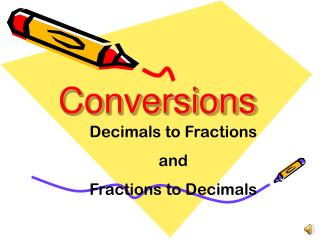DownloadDownload PresentationConversions

# Conversions

Télécharger la présentation## Conversions

- - - - - - - - - - - - - - - - - - - - - - - - - - - E N D - - - - - - - - - - - - - - - - - - - - - - - - - - -
##### Presentation Transcript

1. Conversions Decimals to Fractions and Fractions to Decimals

2. What are we learning? In this lesson you will learn how to convert decimals to fractions and fractions to decimals

3. How to Convert Decimals to Fractions Use the place value of the last digit in the number to determine what the denominator of the fraction will be.

4. How to Convert Decimals to Fractions .5 5 10 The 5 is in the tenths place

5. How to Convert Decimals to Fractions .84 84 100 The 4 is in the hundredths place

6. What if there is a whole number before the decimal point? 1.589 The 9 is in the thousandths place 589 1 1000

7. What if there is a whole number before the decimalpoint? 25.5 The 5 is in the tenths place 5 25 10

8. How to Convert Fractions to Decimals 23 This is the hundredths place so the 3 needs to be in the hundredths place. 100 3 2 .23

9. How to Convert Fractions to Decimals 567 This is the thousandths place so the 7 needs to be in the thousandths place. 1000 6 7 5 .567

10. How to Convert Fractions to Decimals 4 This is the thousandths place so the 4 needs to be in the thousandths place. 1000 0 4 0 .004

11. How to Convert Fractions to Decimals 2 This is the tenths place so the 2 needs to be in the tenths place. 10 2 .2

12. What if there is a whole number before the fraction? 567 This is the thousandths place so the 7 needs to be in the thousandths place. 3 1000 6 7 3 5 3.567

13. How to Convert Fractions to Decimals 34 This is the thousandths place so the 4 needs to be in the thousandths place. 24 1000 24.034

14. Suppose You Can’t Use A Denominator of 10? 5 Divide the Numerator by the Denominator 6

15. Suppose You Can’t Use A Denominator of 10? 5 3 8 . .0 0 6 5 6 4 8 2 0 .83 18 2

16. Suppose You Can’t Use A Denominator of 10? 2 6 . .0 3 2 3 1 8 2 .6

17. Try Some . . .check with a calculator 7 5 6 8 50 10 3 12 3 4 16 40

18. Try some and don’t forget to simplify! .95 .25 .35 .875 .125 .6